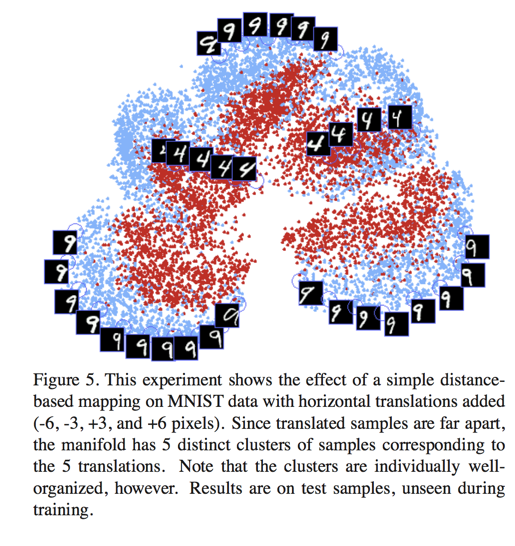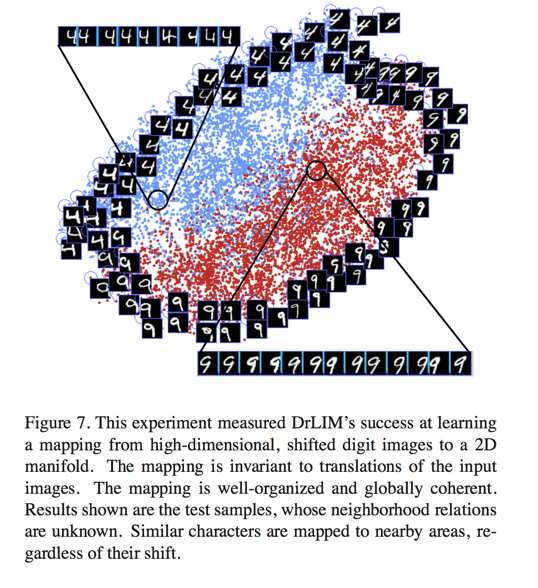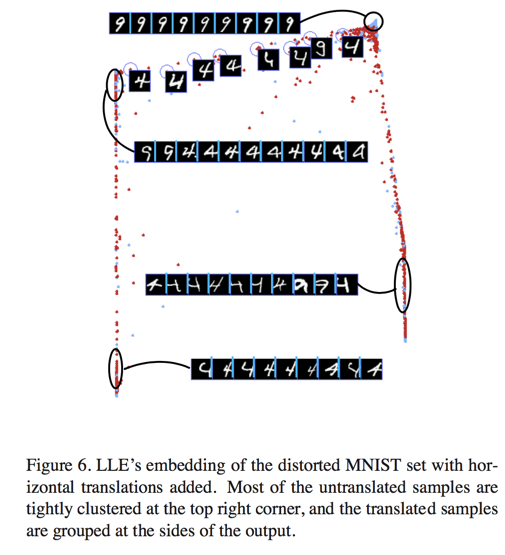# Contrasive Loss(对比损失函数)

Posted by Gwan Siu on June 24, 2017

## 1. What’s the Contrasive Loss function?

Contrasive Loss function is a dimemsion reduction technique. Constrasive loss function can drive the featurs mapping function to learn a mapping that projects high dimension features into its low dimension manifold. In this low dimension manifold, the sample of the same category would cluster together and part away from the sample of different categories.(对比损失函数是一种降维学习方法，它可以学习一种映射关系，这种映射关系可以使得在高维空间中，相同类别但距离较远的点，通过函数映射到低维空间后，距离变近，不同类别但距离都较近的点，通过映射后再低维空间变得更远。这样的结果就是，在低维空间，同一种类的点会产生聚类的效果，不同种类的mean会隔开。类似fisher降维，但fisher降维不具有out-of-sample extension的效果，不能对new sample进行作用)

## 2. Why is contrasive loss funtion needed?

Conventionally, dimension reduction involves mapping a set of high dimensional input points onto a low dimensional manifold so that “similar” points in input space are mapped to nearby points on the manifold. The classical dimension reduction methods are Principle Component Analysis(PCA) and Multi-Dimensional Scaling(MDS). PCA is to project sample points into a subspace where the variance of sample points is minimized. MDS is to project samples into a subspace that is best to preserve the pairwise distances between input points. Another dimension reduction methods are ISOMAP and Local-linear Embedding(LLE). All of above methods presuppose the the existence of a meaningful metric in input space and the computation process usually has three steps: 1.Identify a list of neighborhoods of each points. 2. A gram matrix is computed using this information. 3. the eigenvalue problem is solved for this matrix.

None of these methods attempt to compute a function that could map a new, unknown data point without recomputing the entire embedding and with- out knowing its relationships to the training points.

## 3. What’s the advantage of conrtasive loss function?

1. It only needs neighborhood relationships between training samples. These relationships could come from prior knowledge, or manual labeling, and be indepen- dent of any distance metric.

2. Itmaylearnfunctionsthatareinvarianttocomplicated non-linear trnasformations of the inputs such as light- ing changes and geometric distortions.

3. The learned function can be used to map new samples not seen during training, with no prior knowledge.

4. The mapping generated by the function is in some sense “smooth” and coherent in the output space.

## 4. How does the contrasive loss work?

Let’s consider a pair of sample $\vec{X_{1}},\vec{X_{2}}\in I$ Let $Y$ be a binary label assigned to this pair. $Y = 0$ if $\vec{X_{1}}$ and $\vec{X_{2}}$ are deemd similar, and $Y = 1$ if they are deemed dissimilar. Define the parameterized distance function to be learned DW between $\vec{X_{1}}$, $\vec{X_{2}}$ as the euclidean distance between the outputs of $G_{W}$ . That is:

$D_{W}(\vec{X_{1}},\vec{X_{2}})=\text{||}G_{W}(\vec{X_{1}})-G_{W}(\vec{X_{2}}\text{||}_{2}$

The general form is:

$L(W)=\sum_{i=1}^{P}L(W,(Y,\vec{X}_{1}, \vec{X}_{2})^{i})$ $L(W,(Y,\vec{X_{1}}, \vec{X_{2}})^{i})=(1-Y)L_{S}(D_{W}^{i})+YL_{D}(D^{i}_{W})$

where $(Y,\vec{X}{1}, \vec{X}{2})^{i})$ is the i-th labeled sample pair, $L_{S}$ is the partial loss function for a pair of similar points, $L_{D}$ the partial loss function for a pair of dissimilar points, and P the number of training pairs(which may be as large as the square of the number of samples).

$L_{S}$ and $L_{D}$ must be designed such that minimizing $L$ with respect to $W$ would result in low values of $D_{W}$ for similar pairs and high values of $D_{W}$ for dissimilar pairs.

For example, the exact loss function is:

$L(W,(Y,\vec{X_{1}}, \vec{X_{2}})^{i})=(1-Y)L_{S}(D_{W}^{i})+Y\frac{1}{2}\{\text{max}(0,m-D_{w})\}^{2}$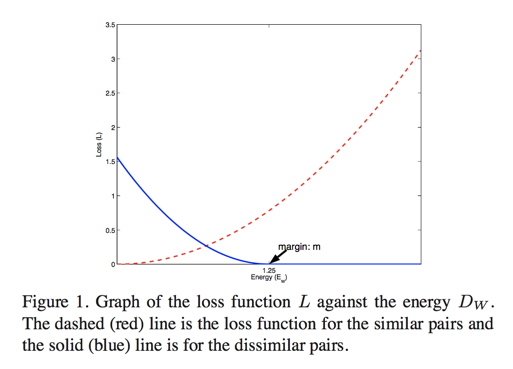where $m>0$ is margin. The margin define a radius around $G_{W}(\vec{X})$. Disimilar pairs contribute to the loss only if their distance is within the his radius.

## 5 Spring model Explaination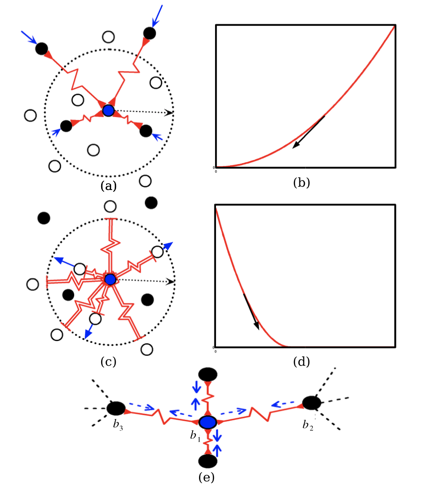Black points are the same category as the blue point while the while points are different category.

## 6 Visual Graph